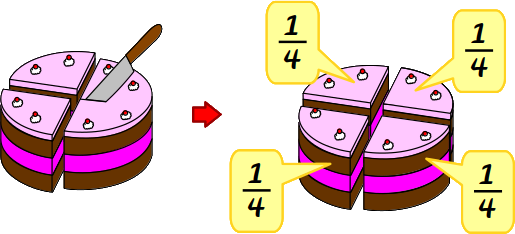# What is a quarter

## quarter

A quarter is created when you divide a whole into four equal parts and take one of these four parts. You can therefore also write a quarter as a fraction: In the denominator (lower number) there is a 4, since it was divided into four parts. There is a 1 in the counter (upper number) because you took a part. It looks like this: ¼. As a decimal you write a quarter of 0.25. That's why you calculate the fraction, i.e. divide 1 by 4: 1: 4 = 0.25.

This is how a quarter is created:That's how it looks:
You have a whole chocolate cake here. It consists of 1 piece.This chocolate cake is now cut in half. This creates 2 pieces of the same size. Each piece represents half of the chocolate cake.These two pieces or halves are now cut again in the middle. This results in 4 pieces of equal size. Each piece represents a quarter of the chocolate cake.Now you take 1 of these quarter pieces. What you have is a quarter of the whole chocolate cake.If you divide a whole into four equal parts and take one of those four parts, you have a quarter.